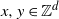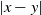Hostname: page-component-594f858ff7-hf9kg Total loading time: 0 Render date: 2023-06-08T18:29:01.768Z Has data issue: false Feature Flags: { "corePageComponentGetUserInfoFromSharedSession": true, "coreDisableEcommerce": false, "corePageComponentUseShareaholicInsteadOfAddThis": true, "coreDisableSocialShare": false, "useRatesEcommerce": true } hasContentIssue false

## Abstract

Scale-free percolation is a stochastic model for complex networks. In this spatial random graph model, vertices$x,y\in\mathbb{Z}^d$ are linked by an edge with probability depending on independent and identically distributed vertex weights and the Euclidean distance$|x-y|$ . Depending on the various parameters involved, we get a rich phase diagram. We study graph distance and compare it to the Euclidean distance of the vertices. Our main attention is on a regime where graph distances are (poly-)logarithmic in the Euclidean distance. We obtain improved bounds on the logarithmic exponents. In the light tail regime, the correct exponent is identified.

## MSC classification

Type
Original Article
Information
Journal of Applied Probability , March 2023 , pp. 295 - 313

## Access options

Get access to the full version of this content by using one of the access options below. (Log in options will check for institutional or personal access. Content may require purchase if you do not have access.)

## References

Benjamini, I., Kesten, H., Peres, Y. and Schramm, O. (2004). Geometry of the uniform spanning forest: Transitions in dimensions$4, 8, 12, \ldots$ Ann. Math. 160, 465491.CrossRefGoogle Scholar
Berger, N. (2004). A lower bound for the chemical distance in sparse long-range percolation models. Preprint, arXiv:math/0409021.Google Scholar
Biskup, M. (2004). On the scaling of the chemical distance in long-range percolation models. Ann. Prob. 32, 29382977.CrossRefGoogle Scholar
Biskup, M. and Lin, J. (2019). Sharp asymptotic for the chemical distance in long-range percolation. Random Structures Algorithms 55, 560583.CrossRefGoogle Scholar
Bringmann, K., Keusch, R. and Lengler, J. (2019). Geometric inhomogeneous random graphs. Theoret. Comput. Sci. 760, 3554.CrossRefGoogle Scholar
Bringmann, K., Keusch, R., Lengler, J., Maus, Y. and Molla, A. R. (2017). Greedy routing and the algorithmic small-world phenomenon. In Proc. 36th ACM Symp. Principles of Distributed Computing. Association for Computing Machinery (ACM), New York, pp. 371–380.CrossRefGoogle Scholar
Bringmann, K., Keusch, R., Lengler, J., Maus, Y. and Molla, A. R. (2022). Greedy routing and the algorithmic small-world phenomenom. J. Comput. Syst. Sci. 125, 59105.CrossRefGoogle Scholar
Dalmau, J. and Salvi, M. (2021). Scale-free percolation in continuum space: Quenched degree and clustering coefficient. J. Appl. Prob. 58, 106127.CrossRefGoogle Scholar
Deijfen, M., van der Hofstad, R. and Hooghiemstra, G. (2013). Scale-free percolation. Ann. Inst. H. Poincaré Prob. Statist. 49, 817838.CrossRefGoogle Scholar
Deprez, P., Hazra, R. S. and Wüthrich, M. V. (2015). Inhomogeneous long-range percolation for real-life network modeling. Risks 3, 123.CrossRefGoogle Scholar
Deprez, P. and Wüthrich, M. (2019). Scale-free percolation in continuum space. Commun. Math. Stat. 7, 269308.CrossRefGoogle Scholar
Ding, J. and Sly, A. (2018). Distances in critical long range percolation. Preprint, arXiv:1303.3995.Google Scholar
Gracar, P., Heydenreich, M., Mönch, C. and Mörters, P. (2022). Recurrence versus transience for weight-dependent random connection models. Electron J. Prob. 27, 1–31.CrossRefGoogle Scholar
Gracar, P., Lüchtrath, L. and Mörters, P. (2021). Percolation phase transition in weight-dependent random connection models. Adv. Appl. Prob. 53, 10901114.CrossRefGoogle Scholar
Heydenreich, M., Hulshof, T. and Jorritsma, J. (2017). Structures in supercritical scale-free percolation. Ann. Appl. Prob. 27, 25692604.CrossRefGoogle Scholar
Hirsch, C. and Mönch, C. (2020). Distances and large deviations in the spatial preferential attachment model. Bernoulli 26, 927947.CrossRefGoogle Scholar
Kleinberg, J. (2000). The small-world phenomenon: An algorithmic perspective. In Proc. 32nd Ann. ACM Symp. Theory of Computing. Association for Computing Machinery, New York, pp. 163–170.CrossRefGoogle Scholar
Milgram, S. (1967). The small world problem. Psychology Today 1, 6167.Google Scholar
Newman, C. M. and Schulman, L. S. (1986). One dimensional$1/|x-y|^s$ percolation models: The existence for a transition for$s\leq 2$ . Commun. Math. Phys. 104, 547571.CrossRefGoogle Scholar
Trapman, P. (2010). The growth of the infinite long-range percolation cluster. Ann. Prob. 38, 15831608.CrossRefGoogle Scholar
van der Hofstad, R. Random Graphs and Complex Networks, Vol. 2 (to appear). Preliminary version available at https://www.win.tue.nl/~rhofstad.Google Scholar
van der Hofstad, R. and Kompathy, J. (2017). Explosion and distances in scale-free percolation. Preprint, arXiv:1706.02597.Google Scholar